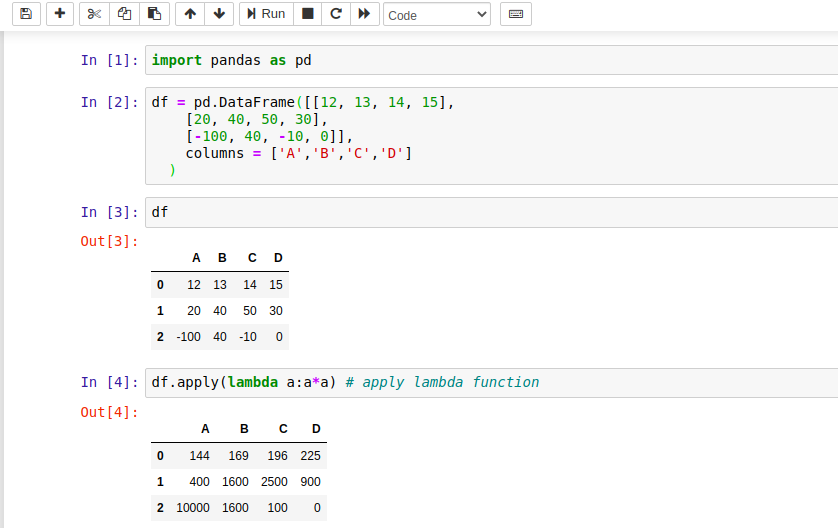# Pandas DataFrame apply() Method

Pandas apply() method is used to apply a function to the DataFrame. The Function can be any valid built-in function or a lambda function.

For example, if we want to get a column-wise or row-wise sum of DataFrame's elements, then we can pass the sum() method of the NumPy library.

This method provides a facility to apply the function on axis bases, means, if we want to apply function for column-wise then set axis = 0, and for row-wise set axis = 1.

Let's understand it by using examples. The syntax of this method is here.

## Syntax

``DataFrame.apply(func, axis=0, raw=False, result_type=None, args=(), **kwds)``

## Parameters

Parameter Type Default Value Required Description
func function No Default Yes Function to apply to each column or row.
axis

{0 or ‘index’, 1 or ‘columns’}, default 0

0 No Axis along which the function is applied:
raw bool False No Determines if row or column is passed as a Series or ndarray object:
result_type {‘expand’, ‘reduce’, ‘broadcast’, None} None No It works when `axis=1` (columns):
args tuple   No

Positional arguments to pass to func in addition to the array/series.

**kwds N/A   No

Additional keyword arguments to pass as keywords arguments to func.

## Return Value

It returns either Series or DataFrame after applying function along the given axis of the DataFrame.

## Example with Jupyter Notebook

Let's take an example to understand the use of pandas.DataFrame.apply() method. Following is the code that is executed by using the Jupyter notebookIf you are familiar with Jupyter, See the screenshot below.## Example: How to Apply function to a DataFrame in Pandas?

Let's apply a lambda function to the DataFrame to get the square of each element. See the example here. This example is similar to the above code that was executed by using the Jupyter notebook. If you are not familiar with Jupyter then see this code to understand.

``````# import pandas library
import pandas as pd
# Create DataFrame of data
df = pd.DataFrame([[12, 13, 14, 15],
[20, 40, 50, 30],
[-100, 40, -10, 0]],
columns = ['A','B','C','D']
)
# print dataframe
print(df)
print("\nApplying apply() method...\n")
# apply() method
df2 = df.apply(lambda a:a*a) # apply lambda function
print(df2)
``````

Output:

A   B    C   D
0   12  13  14  15
1   20  40  50  30
2 -100  40 -10   0

Applying apply() method...

A      B      C     D
0    144   169   196  225
1    400  1600  2500  900
2  10000  1600   100    0

## Example: Apply NumPy's function in Pandas DataFrame

In this example, we are applying the min() function of NumPy to get the min value of each column in the DataFrame. See the example here.

``````# import pandas library
import pandas as pd
import numpy as np
# Create DataFrame of data
df = pd.DataFrame([[12, 13, 14, 15],
[20, 40, 50, 30],
[-100, 40, -10, 0]],
columns = ['A','B','C','D']
)
# print dataframe
print(df)
print("\nApplying apply() method to fetch minimum element\n")
# apply() method
df2 = df.apply(np.min) # find min values from each column
print(df2)
``````

Output:

A    B   C    D
0   12  13  14  15
1   20  40  50  30
2 -100  40 -10   0

Applying apply() method to fetch minimum element

A   -100
B     13
C    -10
D      0
dtype: int64

## Example: Apply function axis-wise to the Pandas DataFrame

By default, the axis parameter value is 0, so the DataFrame apply() function works column-wise. If we wish to apply row-wise function then set axis=1. See the following example here.

``````# import pandas library
import pandas as pd
import numpy as np
# Create DataFrame of data
df = pd.DataFrame([[12, 13, 14, 15],
[20, 40, 50, 30],
[-100, 40, -10, 0]],
columns = ['A','B','C','D']
)
# print dataframe
print(df)
print("\n Fetch minimum element of each column in Dataframe \n")
# apply() method
df2 = df.apply(np.min, axis=0) # find min values from each column
print(df2)
print("\n Fetch minimum element of each row in DataFrame \n")
# apply() method
df2 = df.apply(np.min, axis=1) # find min values from each row
print(df2)
``````

Output:

A   B    C   D
0   12  13  14  15
1   20  40  50  30
2 -100  40 -10   0

Fetch minimum element of each column in Dataframe

A   -100
B     13
C    -10
D      0
dtype: int64

Fetch minimum element of each row in DataFrame

0     12
1     20
2   -100
dtype: int64

## Conclusion

Well, in this topic, we have learned to apply function to the Pandas DataFrame and function can be applied to either column-wise or row-wise.

If we missed something, you can suggest us at - [email protected]

Trending